# Pearson curves

The name of a family of continuous probability distributions (Pearson distributions) whose densities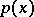satisfy the differential equation(*)

where the parameters,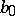,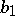, andare real numbers. More precisely, Pearson curves are graphs ofas a function of. The distributions that are solutions to (*) coincide with limiting forms of the hypergeometric distribution. Pearson curves are classified in accordance with the character of the roots of the equation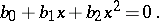The family of Pearson curves consists of 12 types plus the normal distribution. Many very important distributions in mathematical statistics may be obtained by a transformation from (*).

W. Elderton (1938) gave a systematic description of the types of Pearson curves. In simplified form, the classification by types is as follows:

Type I: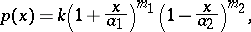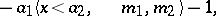with as a particular case the beta-distribution of the first kind.

Type II: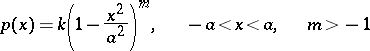(a version of a type-I Pearson curve); a particular case is the uniform distribution.

Type III: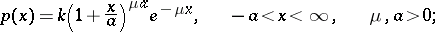particular cases are the gamma-distribution and the "chi-squared" distribution.

Type IV: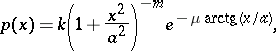Type V:(which can be reduced by transformation to type III).

Type VI: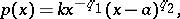particular cases are the beta-distribution of the second kind and the Fisher-distribution.

Type VII: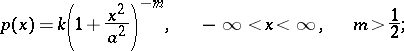a particular case is the Student distribution.

Type VIII:Type IX: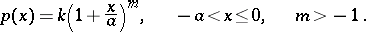Type X:i.e. an exponential distribution.

Type XI: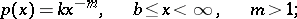a particular case is the Pareto distribution.

Type XII: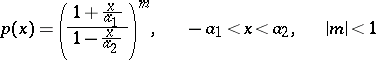(a version of Type I).

The most important distributions for applications are the Types I, III, VI, and VII.

Any Pearson curve is uniquely determined by the first four of its moments: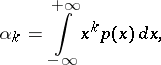if these are finite. This property of the family of Pearson curves is used for the approximate description of empirical distributions (cf. Empirical distribution).

The method of fitting Pearson curves to some empirical distribution is as follows. Independent observations are used to calculate the first four sample moments, and then the suitable type of Pearson curve is determined, using the method of moments to find the unknown parameters. In the general case, the method of moments is not an efficient method for obtaining estimators of Pearson curves. L.N. Bol'shev (1963) provided a new solution to the problem of a more accurate approximation to distributions by means of Pearson curves using asymptotic transformations.

The curves were introduced by K. Pearson in 1894.